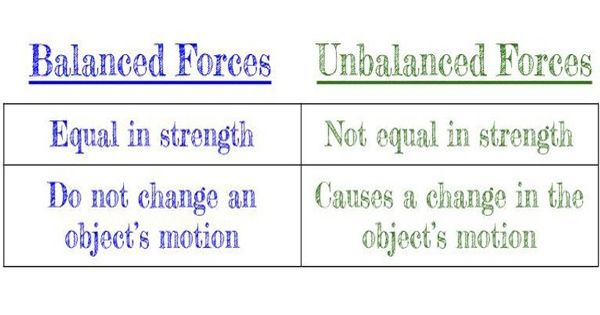Physics

# Difference between Balanced and Unbalanced ForcesForces have a magnitude (strength) and a direction. Balanced forces are equal in size and opposite in direction. It implies the force which does not change the object’s state of motion. When forces are balanced, there is no change in motion. On the contrary, unbalanced forces are the one that results in the change in object’s state of motion. This happens in two ways. If an object is at rest and an unbalanced force pushes or pulls the object, it will move.

Balanced forces will cause no change in the speed of an object. Unbalanced forces are not equal, and they always cause the motion of an object to change the speed and/or direction that it is moving.

In balanced forces, the two individual forces act in opposite direction. Conversely, in unbalanced forces, the individual forces either act in same or opposite direction.

Difference between Balanced and Unbalanced Forces

BALANCED FORCES

• When the forces exerted on the object, are of equal size but in opposite direction, then the forces are known as balanced forces.
• When the individual forces applied on an object are of same magnitude and opposite direction, then the forces are known as balanced forces.
• Balanced Forces, as the name suggest are the forces that balance each other, when acted upon an object, causing the object to maintain its equilibrium and does not accelerate. If the forces that are applied to the object are of equal magnitude but are opposite in direction, the forces are said to be balanced.
• Balanced forces cause a still object to remain at rest.
• When the balanced forces are applied to a stationary object, it stays still, but when it is applied on a moving object, it continues moving with a constant speed and same direction.
• If the object is in motion and balanced forces are applied, then the object will continue to move with the same speed.

UNBALANCED FORCES

• When the forces applied on the object are unequal in size, then the forces are known as unbalanced forces.
• When two unbalanced forces are exerted in opposite directions, their combined force is equal to the difference between the two forces.
• The force which is not counterbalanced by a force of equal magnitude and diametrically opposite direction, resulting in disequilibrium of the object and eventually accelerates, it is known as unbalanced forces.
• The unbalanced forces cause a stationary object to move in the direction of the greater force.
• In unbalanced forces, the net force will be non-zero, and the object will move in the direction of the greater force. Thus, it causes acceleration in the object, i.e. stationary objects move, moving objects speed up, slow down, stop or change their direction of motion.
• If unbalanced forces are exerted on a moving object then it will slow down, speed up, stop, or its direction will be changed.

Information Source: